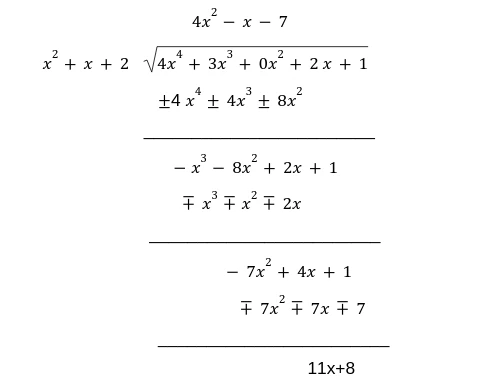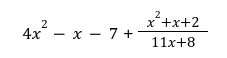# What is the Concept of the Polynomial Long Division?It is a method of solving the long division of a number into steps to make it easy for the students to learn. There are different online tools that make the whole procedure easy for the students. Polynomial Long Division Calculator highlights the procedure of the long division as simple and amazingly easy to solve. In this process, it is essential for you to understand the different patterns in the long division problems: the dividend and the figure divided by the divisor.

The result of the long decision problem is the quotient and if something remains after the long decision is the remainder of the long division. The long division concept is essential to understand students, as it is one of the basic concepts along with addition, subtraction, and multiplication. If you are able to understand the long division method of the number. Then you can apply the same procedure for doing the polynomials.

## What is the Concept of Long Division?

The basic concepts of division, multiplication, and addition are similar. When we are applying these concepts to algebra. Then we have to follow the same concepts. Using online technology helps to understand the various steps of the long division in detail. It actually reveals what are the various steps in solving the long division method. The polynomial long division calculator can be used to find the long division of the polynomials.

## Practical Example:

Consider we have a numerator "4x4 + 3x3 + 0x2 + 2x + 1" and the denominator, x2+x+2. Now by applying the polynomial long division calculator, the remainder we are getting 11x+15.Now we can write it asIt can be difficult to solve the long division of the polynomial, but when you are b;e to use the polynomial long division calculator. You can find the answer to the polynomial long division. The main reason for doing the polynomial long division is that we are not familiar with the concept of the quotient, Numerator, and Denominator. When we are able to understand the concept then it would become easy for you.

## How to Make the Concept Easy?

Once you have the basic concepts of the terminologies then it can become easy for you to understand the concept of the long division. Long division polynomial calculator is an online tool that verifies all the steps in detail for the students, and it makes the long-division process fun to solve.

The Polynomial problems are easy to solve if you have learned how to solve the long design of the huge number, as we are following the same steps as for the long division of the polynomial. In arithmetics, long division is the standard division algorithm for dividing large numbers and breaking them down into steps. Use the Long division polynomials calculator for doing the division in the steps.

## Conclusion:

Consider the long division as the regular division problem, the dividend is divided by the divisor which gives a result known as the quotient, and sometimes it gives a remainder too. You may need to use the remainder calculator to find the remainder of the polynomial long division. The whole procedure of the polynomial long division is easy but a little lengthy. Treat the long division of the polynomial by the procedure described in the article.